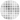# Heatmaps with various input formats

This post explains how to make heatmaps using seaborn. Three main types of input exist to plot a heatmap: wide format, correlation matrix, and long format.

## Wide Format (Untidy)

The wide format (or the untidy format) is a matrix where each row is an individual, and each column is an observation. In this case, the heatmap makes a visual representation of the matrix: each square of the heatmap represents a cell. The color of the cell changes according to its value. In order to draw a heatmap with a wide format dataset, you can use the `heatmap()` function of seaborn.

``````# library
import seaborn as sns
import pandas as pd
import numpy as np

# Create a dataset
df = pd.DataFrame(np.random.random((5,5)), columns=["a","b","c","d","e"])

# Default heatmap: just a visualization of this square matrix
sns.heatmap(df)``````
``<AxesSubplot:>``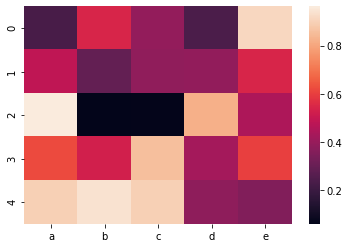## Correlation Matrix (Square)

Suppose you measured several variables for n individuals. A common task is to check if some variables are correlated. You can easily calculate the correlation between each pair of variable, and plot this as a heatmap. This lets you discover which variable is related to the other.

As a difference from the previous example, you will give a correlation matrix as an input instead of a wide format data.

``````# library
import seaborn as sns
import pandas as pd
import numpy as np

# Create a dataset
df = pd.DataFrame(np.random.random((100,5)), columns=["a","b","c","d","e"])

# Calculate correlation between each pair of variable
corr_matrix=df.corr()

# plot it
sns.heatmap(corr_matrix, cmap='PuOr')``````
``<AxesSubplot:>``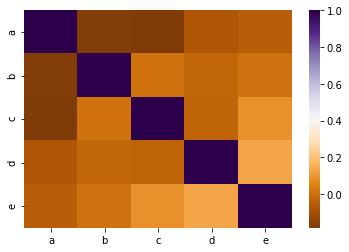Note that in this case, both correlations (i.e. from a to b and from b to a) will appear in the heatmap. You might want to plot a half of the heatmap using `mask` argument like this example:

``````# library
import seaborn as sns
import pandas as pd
import numpy as np
np.random.seed(0)

# Create a dataset
df = pd.DataFrame(np.random.random((100,5)), columns=["a","b","c","d","e"])

# Calculate correlation between each pair of variable
corr_matrix=df.corr()

# Can be great to plot only a half matrix
# Generate a mask for the upper triangle

# Draw the heatmap with the mask
``````
``<AxesSubplot:>``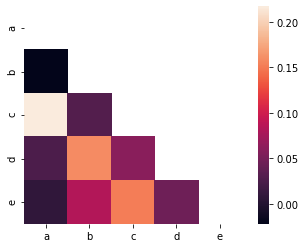## Long Format (Tidy)

In the tidy or long format, each line represents an observation. You have 3 columns: individual, variable name, and value (x, y and z). You can plot a heatmap from this kind of data as follow:

``````# library
import seaborn as sns
import pandas as pd
import numpy as np

# Create long format
people = np.repeat(("A","B","C","D","E"),5)
feature = list(range(1,6))*5
value = np.random.random(25)
df = pd.DataFrame({'feature': feature, 'people': people, 'value': value })

# Turn long format into a wide format
df_wide = df.pivot_table( index='people', columns='feature', values='value')

# plot it
sns.heatmap(df_wide)
``````
``<AxesSubplot:xlabel='feature', ylabel='people'>``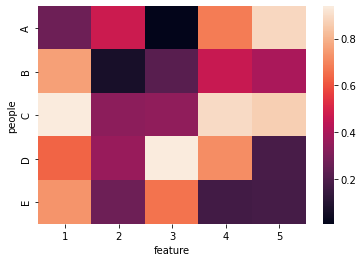## Contact & Edit

👋 This document is a work by Yan Holtz. Any feedback is highly encouraged. You can fill an issue on Github, drop me a message onTwitter, or send an email pasting `yan.holtz.data` with `gmail.com`.